DeepFashion2 团队基于 DeepFashion2 数据集构建了一个统一的分析模型. 很是强大，把服装主体检测、服装关键点估计、服装分割、服装检索做到了 end-to-end，厉害!

 - 服装主体检测：旨在预测图片中的服装边界框及对应的服装类目标签. 评价标准采用 COCO 的 $AP_{box}$、$AP_{box}^{IoU=0.50}$ 和 $AP_{box}^{IoU=0.75}$.

 - 服装关键点检测：旨在预测图片中每个检测到的服装主体的关键点位置. 评价标准采用 COCO human pose estimation 的 $AP_{pt}$、$AP_{pt}^{OKS=0.50}$ 和 $AP_{pt}^{OKS=0.75}$，其中 $OKS$ 表示物体关键点的相似性.

 - 服装分割： 旨在对图片中每个服装主体的每一像素进行分类(包括 background). 评价标准采用 $AP_{mask}$、$AP_{mask}^{IoU=0.50}$ 和 $AP_{mask}^{IoU=0.75}$.

 - 服装检索(Commercial-Consumer)：给定 Consumer 拍摄的图片检测到的服装，搜索与之对应的相似的 Commercial 商品图片. 评价标准采用 top-k 检索精度. 这里会考虑服装检测器的影响，如果没有检测到服装主体，则查询的商品也是缺失的. 具体的，在 DeepFashion2 traning 数据集中共有 686K 组 commercial-consumer 服装对；在 validation 数据集中，共有 10990 张 consumer 图片，其包含 12550 个服装主体作为待查询数据集，21436 张 commercial 图片，其包含 37183 个服装主体作为查询数据集；在 test 数据集中，共有 21550 张 consumer 图片，其包含 24402 个服装主体作为待查询数据集，43608 张 commercial 图片，其包含 75347 个服装主体作为查询数据集.

## 1. Match R-CNN

Match R-CNN 如图：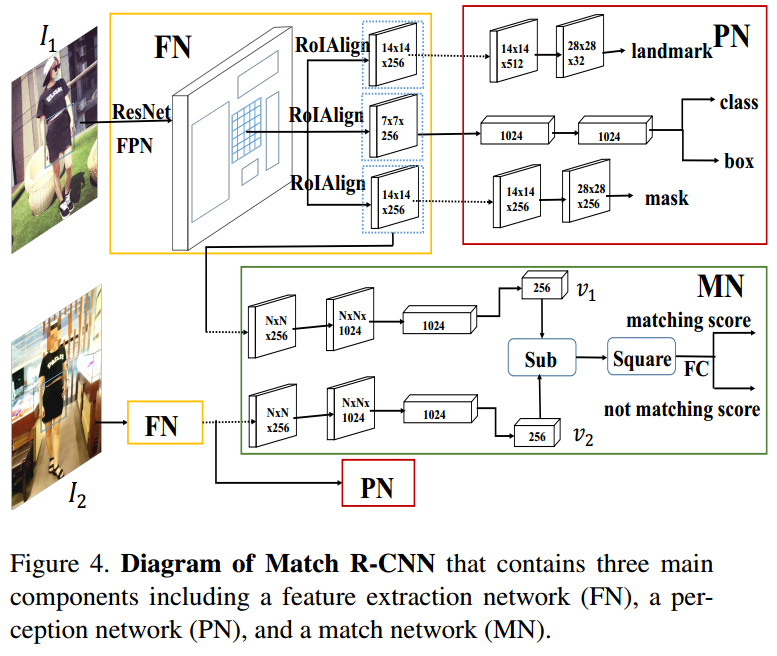### 1.1. Match R-CNN 流程

Match R-CNN 采用两张图片 $I_1$ 和 $I_2$ 作为输入.

 - Stage1 - Feature Network(FN)

FN 由 ResNet-FPN backbone、RPN(region proposal network) 和 RoI Align 模块组成.

 - Stage2 - Perception Network(PN)

Stage1 中 FN 提取的 RoI 特征被分别送入 PN 的三个网络分支.

 - Stage3 - Matching Network(MN)

MN 包含一个特征提取网络和一个用于服装检索的相似性学习网络.

### 1.2. Loss 函数

Match R-CNN 的参数 $\Theta$ 的优化，是通过最小化五个损失函数来实现的，即：

$$\mathcal{L} = \lambda_1 \mathcal{L}_{cls} + \lambda_2 \mathcal{L}_{box} + \lambda_3 \mathcal{L}_{pose} + \lambda_4 \mathcal{L}_{mask} + \lambda_5 \mathcal{L}_{pair}$$

 - $\mathcal{L}_{cls}$ 采用交叉熵(CE) loss，用于服装分类；

 - $\mathcal{L}_{box}$ 采用 smooth loss，用于边界框回归；

 - $\mathcal{L}_{pose}$ 采用交叉熵(CE) loss，用于关键点估计；

 - $\mathcal{L}_{mask}$ 采用交叉熵(CE) loss，用于服装分割；

 - $\mathcal{L}_{pair}$ 采用交叉熵(CE) loss，用于服装检索，具体如下：

$$\mathcal{L}_{pair} = - \frac{1}{n} \sum_{i=1}^n [y_i log(\hat{y}_i) + (1-y_i)log(1 - \hat{y}_i)]$$

### 1.3. 实现

 - 每张训练图片 resize，保持其最短边为 800 pixels，最长边不超过 1333 pixels.

 - 每个 minibatch 中，单张 GPU 处理两张图片，共采用 8 块 GPU 进行训练.

 - minibatch size 为 16，初始化学习率 LR=0.02，并在 8 个 epochs、11 个 epochs 后以 0.1 的因子衰减 LR，训练 12 个 epochs 后停止. 记为 1x.

### 1.4. 推断Inference

top-1000 proposals 检测结果用于边界框分类与边界框回归. 然后，对这些 proposals 进行 NMS 过滤处理，再分别送入关键点估计分支和 mask 分割分支.

## 2. Results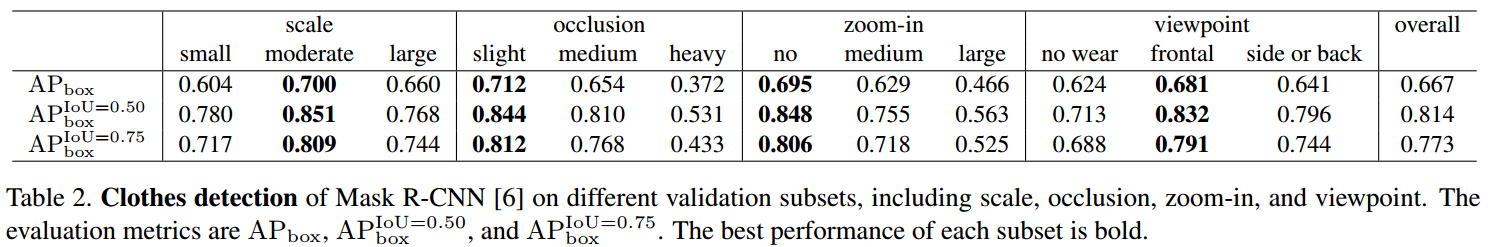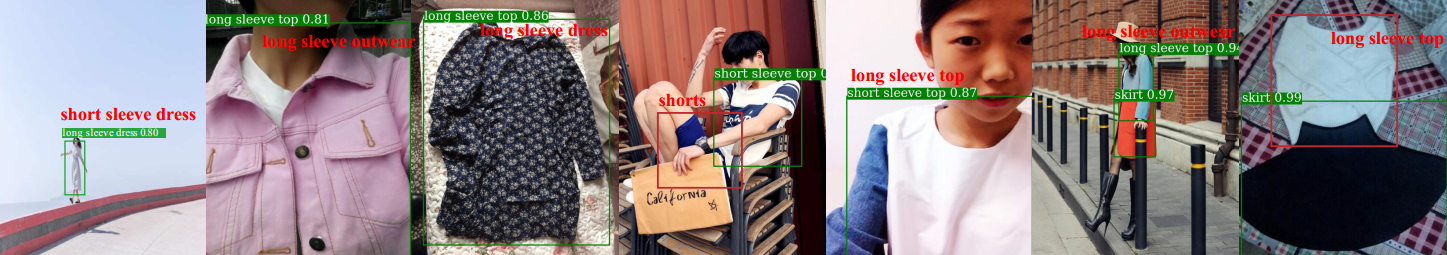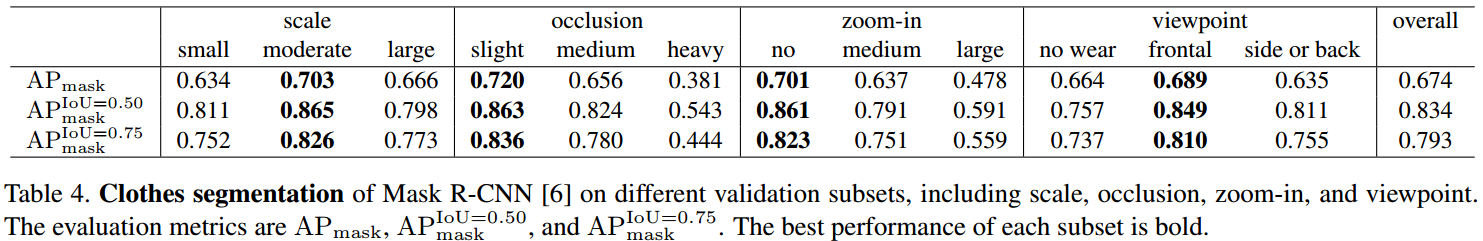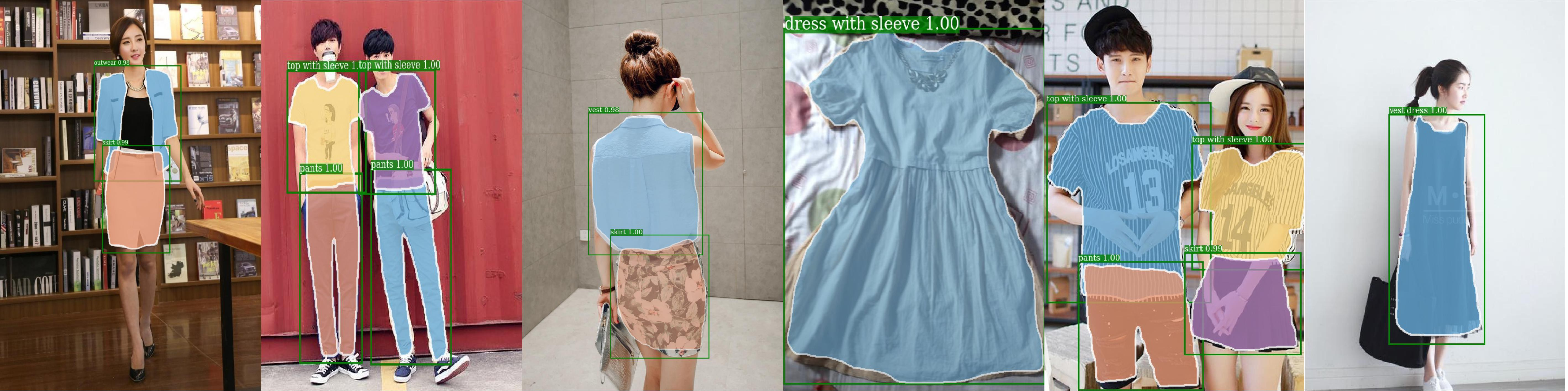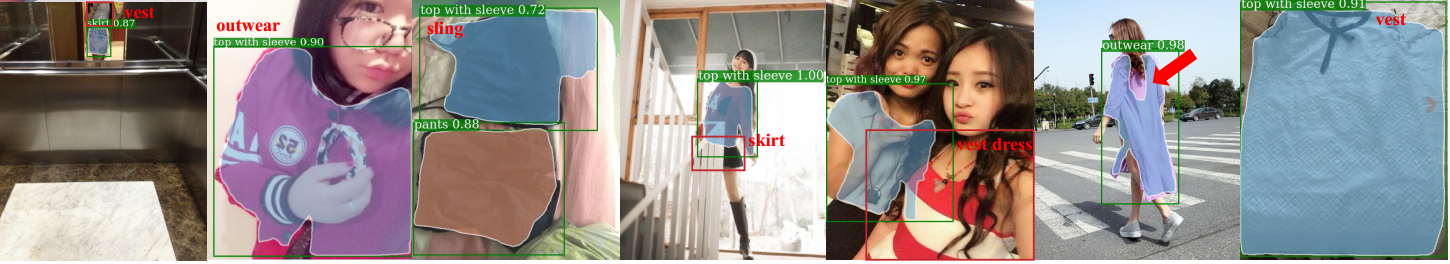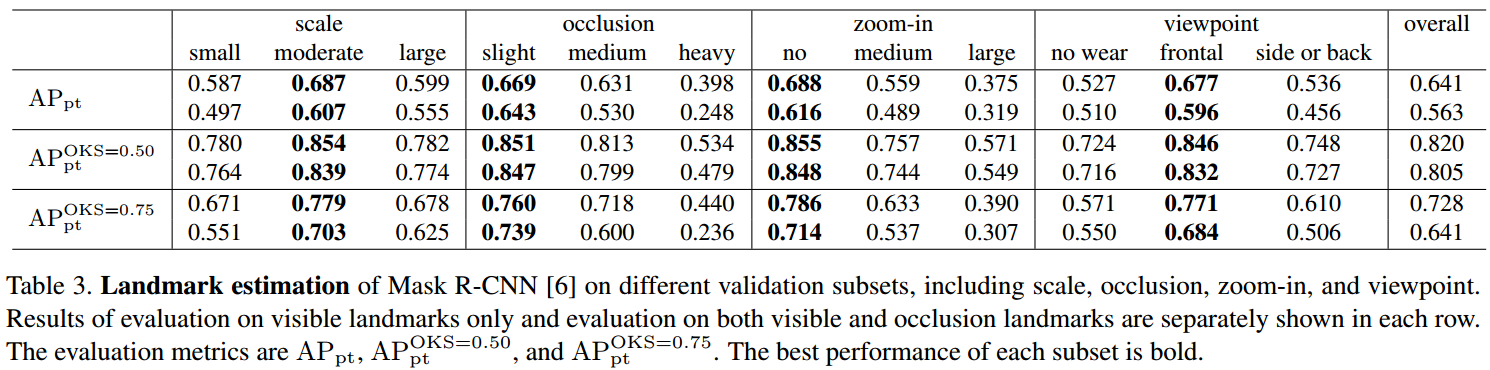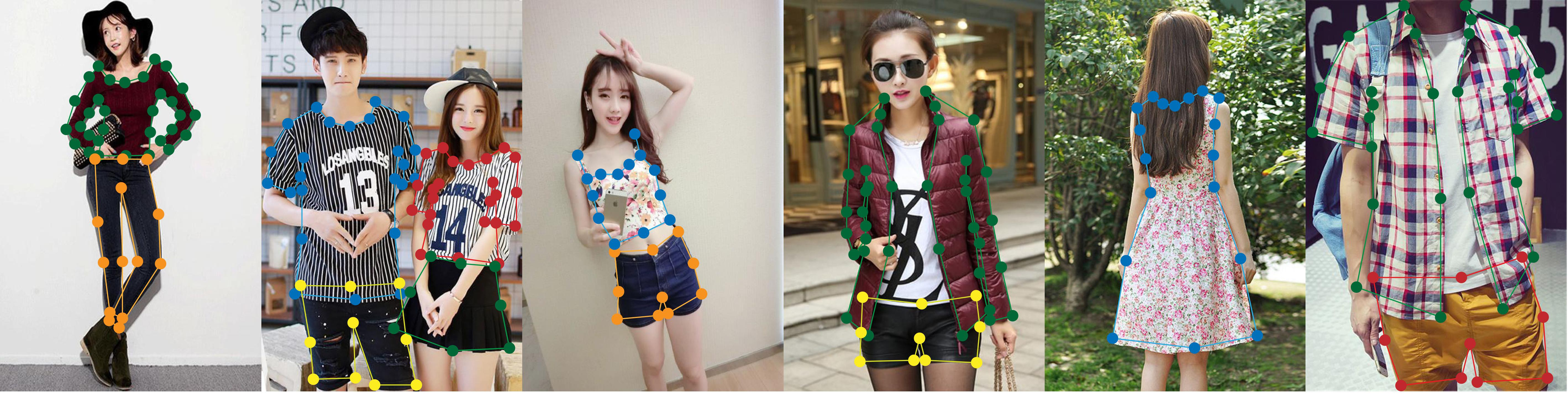### 2.4. Match R-CNN 服装检索

Consumer-to-Shop Clothes Retrieval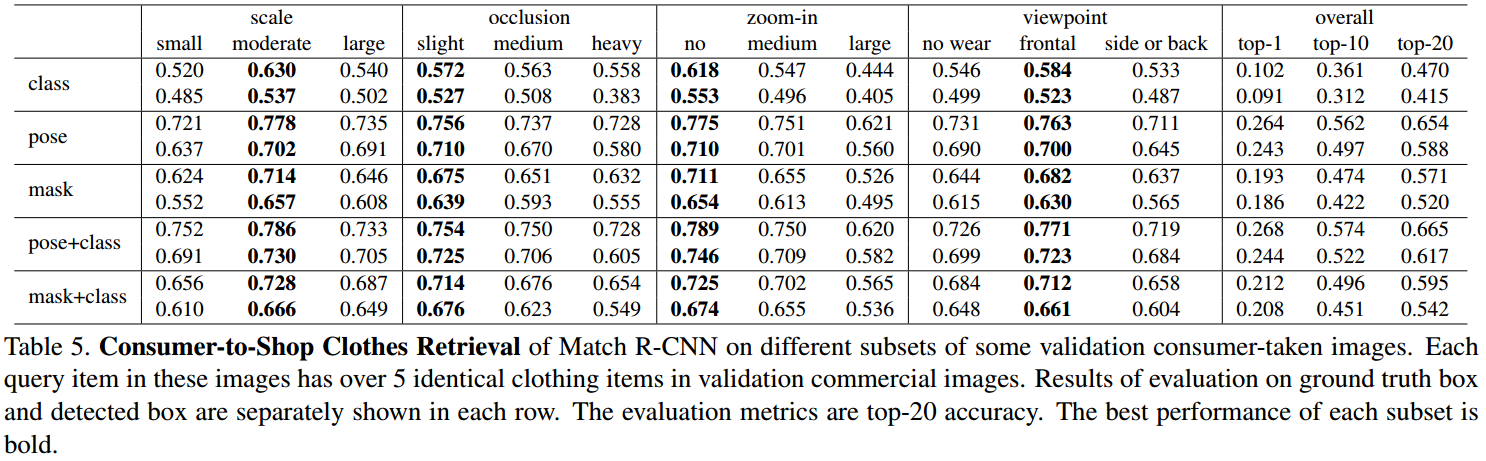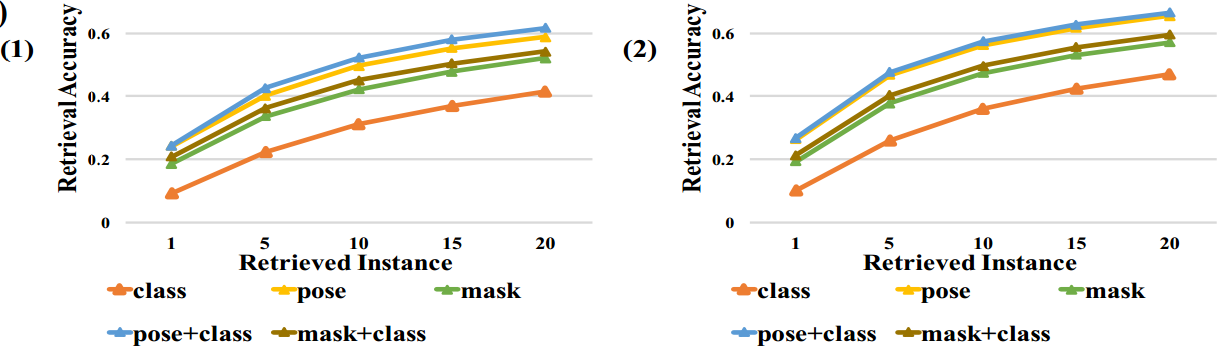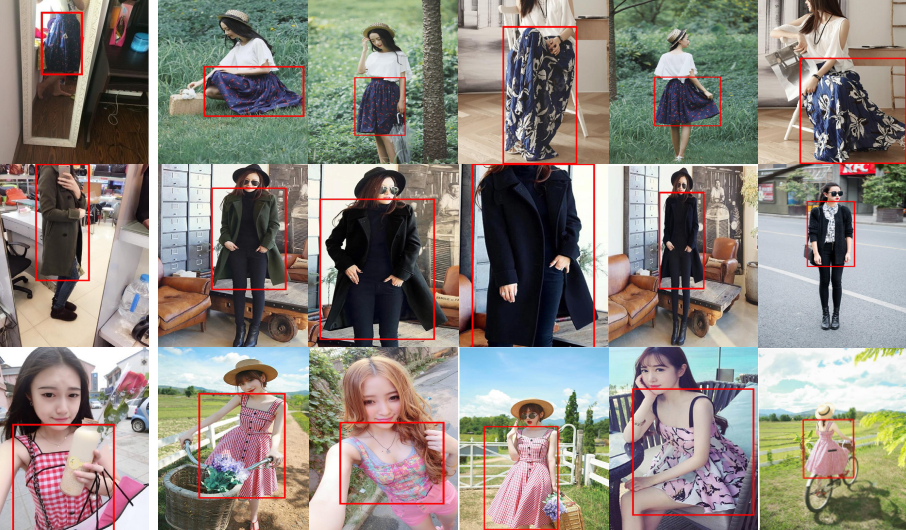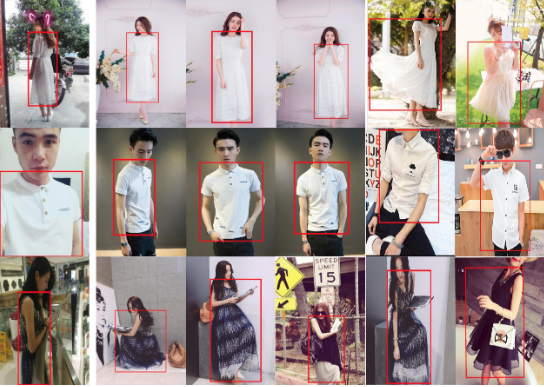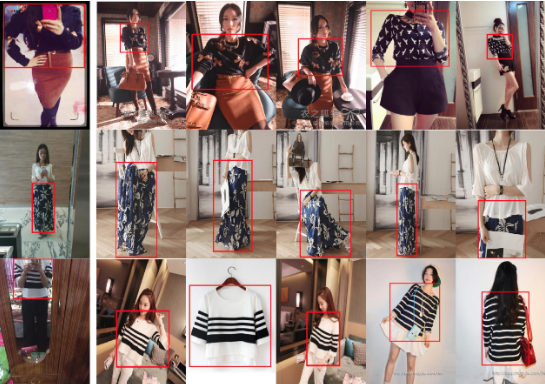Last modification：June 20th, 2019 at 11:06 am